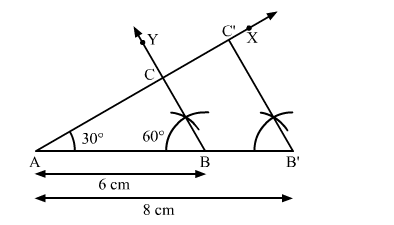# Construct a ∆ABC in which AB = 6 cm, ∠A = 30º and ∠B = 60º.`
Question:

Construct a $\triangle \mathrm{ABC}$ in which $\mathrm{AB}=6 \mathrm{~cm}, \angle \mathrm{A}=30^{\circ}$ and $\angle \mathrm{B}=60^{\circ} .$ Construct another $\Delta \mathrm{AB}^{\prime} \mathrm{C}^{\prime}$ similar to $\Delta \mathrm{ABC}$ with base $\mathrm{AB}^{\prime}=8 \mathrm{~cm}$.

Solution:

Steps of Construction

Step 1. Draw a line segment AB = 6 cm.

Step 2. At A, draw ">XAB = 30º.

Step 3. At B, draw ">YBA = 60º. Suppose AX and BY intersect at C.

Thus, ∆ABC is the required triangle.

Step 4. Produce AB to B' such that AB' = 8 cm.

Step 5. From B', draw B'C' || BC meeting AX at C'.Here, ∆AB'C' is the required triangle similar to ∆ABC.